Next: EM Algorithm Up: Algorithms for Principal Component Previous: Principal subspace and components

Singular Value Decomposition

PCA can be determined by using the singular value decomposition (SVD)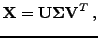(3)

where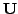is a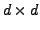orthogonal matrix,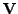is an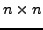orthogonal matrix and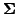is a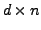pseudodiagonal matrix (diagonal if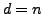) with the singular values on the main diagonal . The PCA solution is obtained by selecting the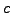largest singular values from, by forming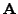from the correspondingcolumns of, and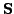from the correspondingrows of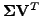.

Note that PCA can equivalently be defined using the eigendecomposition of thecovariance matrix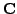of the column vectors of the data matrix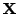: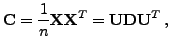(4)

Here, the diagonal matrix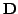contains the eigenvalues of, and the columns of the matrixcontain the unit-length eigenvectors ofin the same order [6,4,2,5]. Again, the columns ofcorresponding to the largest eigenvalues are taken as, andis computed as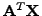. This approach can be more efficient for cases where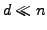, since it avoids thematrix.Next: EM Algorithm Up: Algorithms for Principal Component Previous: Principal subspace and components
Tapani Raiko 2007-09-11